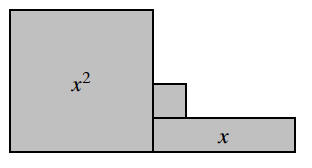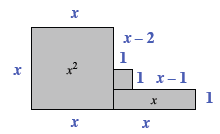### Home > CC2 > Chapter 5 > Lesson 5.3.2 > Problem5-114

5-114.Look at the algebra tile shape at right.

1. Write an algebraic expression for the perimeter of the shape in two ways: first, by finding the length of each of the sides and adding them all together; second, by writing an equivalent, simplified expression.

Label all the side lengths.

$x+x+x-2+1+1+x-1+1+x+x$

Simplify this expression.2. Write an algebraic expression for the area of the shape.

To find the area, add up the values of all the tiles within the perimeter.